## Evelyn is taking a multiple choice test with a total of 20 points available. Each question is worth exactly 1 point. What would be Eve

Question

Evelyn is taking a multiple choice test with a total of 20 points available. Each
question is worth exactly 1 point. What would be Evelyn’s test score (out of 20) if she
got 3 questions wrong? What would be her score if she got x questions wrong?

in progress 0
2 weeks 2021-08-30T03:35:57+00:00 1 Answers 0 views 0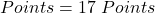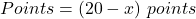Step-by-step explanation:

Given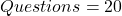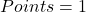Solving (a): 3 questions wrong

First, we need to determine the total possible points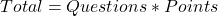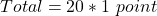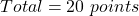— (1)

Next, is to determine the points of 3 questions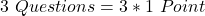— (2)

Subtract (2) from (1)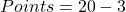Her test score is 17 points

Solving (b): x questions wrong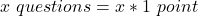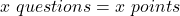— (3)

Subtract (3) from (1)Her points would be 20 – x points